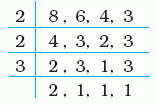Email us to get an instant 20% discount on highly effective K-12 Math & English kwizNET Programs!

#### Online Quiz (WorksheetABCD)

Questions Per Quiz = 2 4 6 8 10

### MEAP Preparation - Grade 6 Mathematics2.30 Prime Factorization and LCM

 Method 2: Write the given numbers in a row. Think of a prime number that will divide atleast two of these numbers. We perform the division by writing the quotient in the next row. If a number is not divisible by the prime factor, we copy it to the next row. Example: Find the LCM of 8, 6, 4, 3.LCM = 2 x 2 x 3 x 2 Answer: 24 Directions: Find the LCM using the division method. Also write at least 5 examples of your own.
 Q 1: Find the LCM of 112, 168, 266 by division method.630063846380 Q 2: Find the LCM of 16, 20, 24 by division method.260240250 Q 3: Find by prime factorization the LCM of the following numbers 8, 12, 16404248 Q 4: Find the LCM of 16, 24, 15 by division method.245250240 Q 5: Find by prime factorization the LCM of the following numbers 12, 15, 18160120180 Q 6: Find by prime factorization the LCM of the following numbers 10, 15, 20506054 Q 7: Find the LCM of 6, 8, 10, 12, 14 by division method.870850840 Q 8: Find by prime factorization the LCM of the following numbers 4, 5, 6605650 Question 9: This question is available to subscribers only! Question 10: This question is available to subscribers only!